Anúncio

# ACCA F9 Investment appraisal-Discounted Cash Flow Techniques

20 de Mar de 2019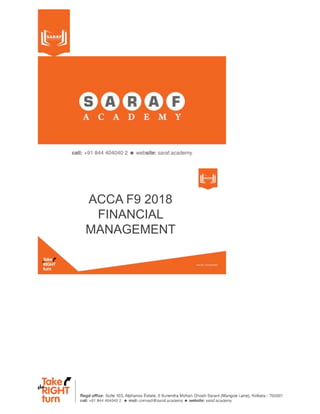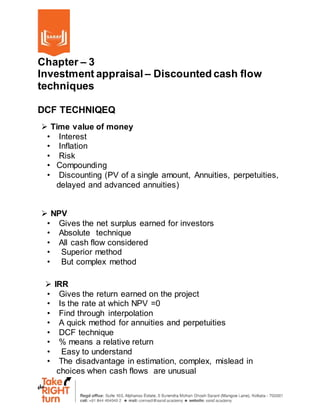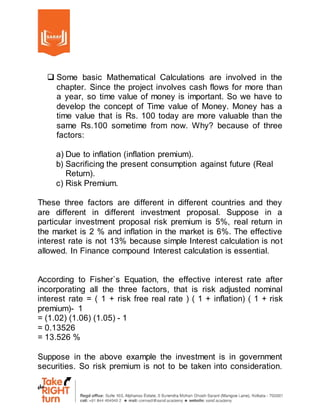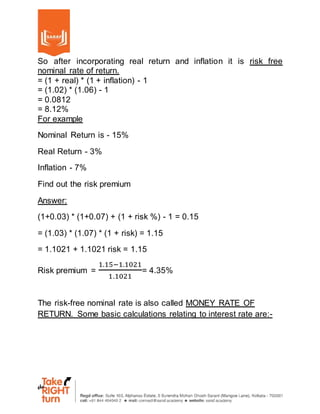Anúncio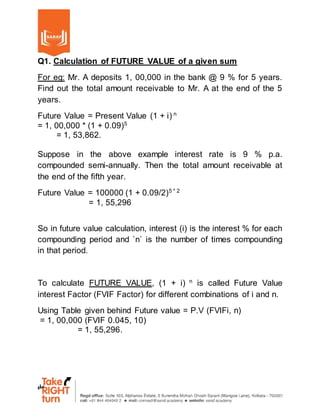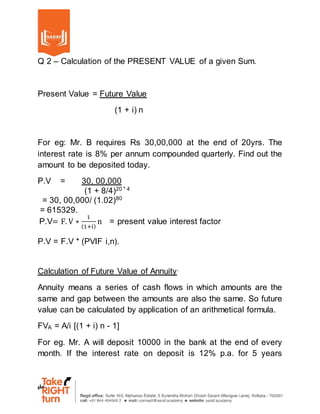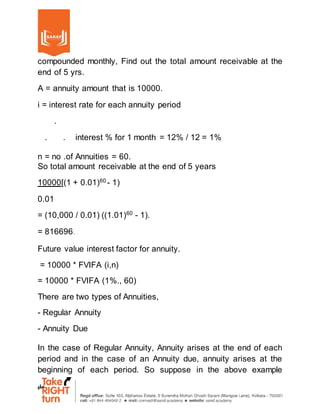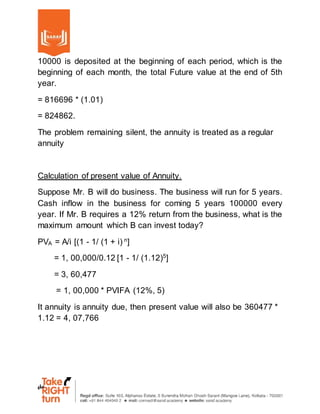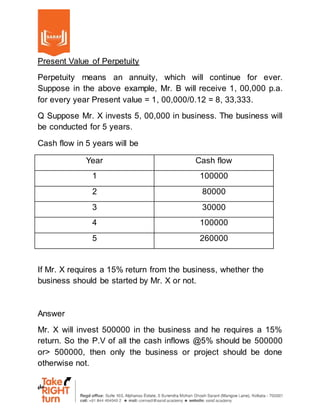Anúncio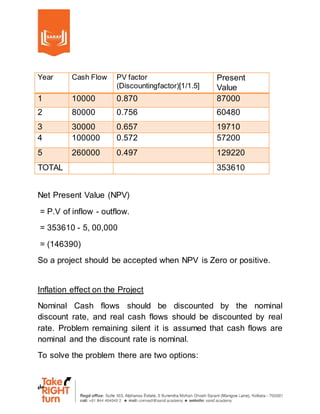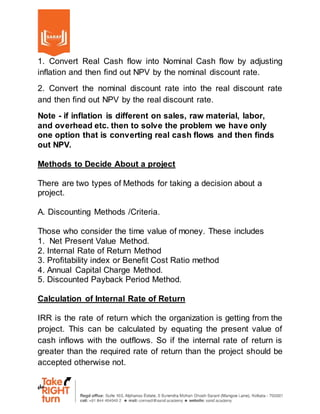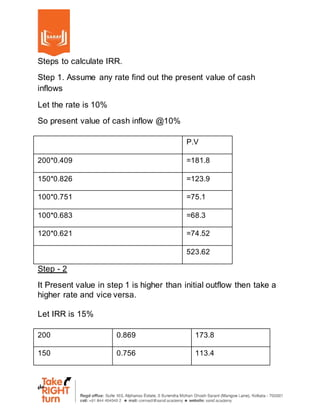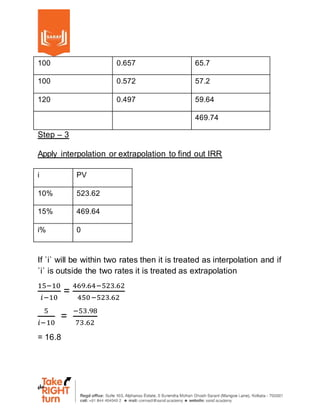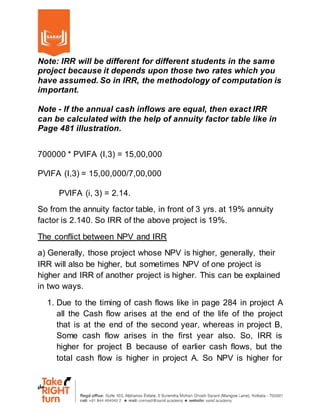Anúncio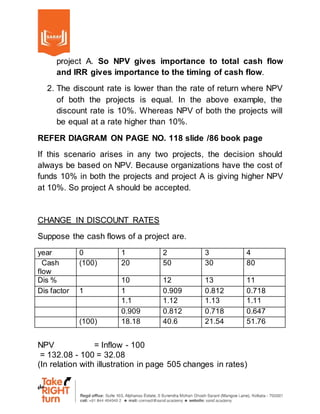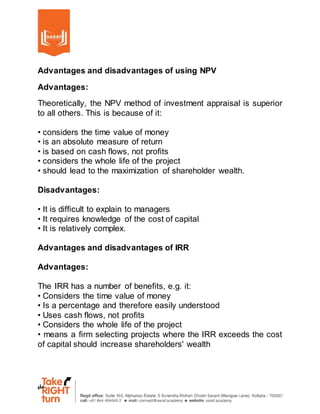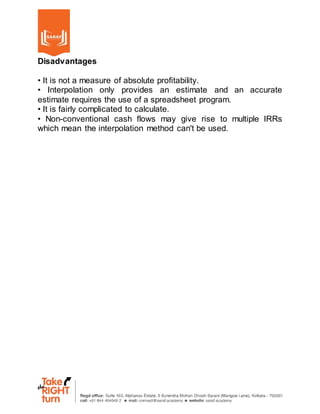Próximos SlideSharesTime Value Of Money
Carregando em ... 3
1 de 17
Anúncio

### ACCA F9 Investment appraisal-Discounted Cash Flow Techniques

1. ACCA F9 2018 FINANCIAL MANAGEMENT
2. Chapter – 3 Investment appraisal – Discounted cash flow techniques DCF TECHNIQEQ  Time value of money • Interest • Inflation • Risk • Compounding • Discounting (PV of a single amount, Annuities, perpetuities, delayed and advanced annuities)  NPV • Gives the net surplus earned for investors • Absolute technique • All cash flow considered • Superior method • But complex method  IRR • Gives the return earned on the project • Is the rate at which NPV =0 • Find through interpolation • A quick method for annuities and perpetuities • DCF technique • % means a relative return • Easy to understand • The disadvantage in estimation, complex, mislead in choices when cash flows are unusual
3.  Some basic Mathematical Calculations are involved in the chapter. Since the project involves cash flows for more than a year, so time value of money is important. So we have to develop the concept of Time value of Money. Money has a time value that is Rs. 100 today are more valuable than the same Rs.100 sometime from now. Why? because of three factors: a) Due to inflation (inflation premium). b) Sacrificing the present consumption against future (Real Return). c) Risk Premium. These three factors are different in different countries and they are different in different investment proposal. Suppose in a particular investment proposal risk premium is 5%, real return in the market is 2 % and inflation in the market is 6%. The effective interest rate is not 13% because simple Interest calculation is not allowed. In Finance compound Interest calculation is essential. According to Fisher`s Equation, the effective interest rate after incorporating all the three factors, that is risk adjusted nominal interest rate = ( 1 + risk free real rate ) ( 1 + inflation) ( 1 + risk premium)- 1 = (1.02) (1.06) (1.05) - 1 = 0.13526 = 13.526 % Suppose in the above example the investment is in government securities. So risk premium is not to be taken into consideration.
4. So after incorporating real return and inflation it is risk free nominal rate of return. = (1 + real) * (1 + inflation) - 1 = (1.02) * (1.06) - 1 = 0.0812 = 8.12% For example Nominal Return is - 15% Real Return - 3% Inflation - 7% Find out the risk premium Answer: (1+0.03) * (1+0.07) + (1 + risk %) - 1 = 0.15 = (1.03) * (1.07) * (1 + risk) = 1.15 = 1.1021 + 1.1021 risk = 1.15 Risk premium = 1.15−1.1021 1.1021 = 4.35% The risk-free nominal rate is also called MONEY RATE OF RETURN. Some basic calculations relating to interest rate are:-
5. Q1. Calculation of FUTURE VALUE of a given sum For eg: Mr. A deposits 1, 00,000 in the bank @ 9 % for 5 years. Find out the total amount receivable to Mr. A at the end of the 5 years. Future Value = Present Value (1 + i) n = 1, 00,000 * (1 + 0.09)5 = 1, 53,862. Suppose in the above example interest rate is 9 % p.a. compounded semi-annually. Then the total amount receivable at the end of the fifth year. Future Value = 100000 (1 + 0.09/2)5 * 2 = 1, 55,296 So in future value calculation, interest (i) is the interest % for each compounding period and `n` is the number of times compounding in that period. To calculate FUTURE VALUE, (1 + i) n is called Future Value interest Factor (FVIF Factor) for different combinations of i and n. Using Table given behind Future value = P.V (FVIFi, n) = 1, 00,000 (FVIF 0.045, 10) = 1, 55,296.
6. Q 2 – Calculation of the PRESENT VALUE of a given Sum. Present Value = Future Value (1 + i) n For eg: Mr. B requires Rs 30,00,000 at the end of 20yrs. The interest rate is 8% per annum compounded quarterly. Find out the amount to be deposited today. P.V = 30, 00,000 (1 + 8/4)20 * 4 = 30, 00,000/ (1.02)80 = 615329. P.V= F. V ∗ 1 (1+i) n = present value interest factor P.V = F.V * (PVIF i,n). Calculation of Future Value of Annuity. Annuity means a series of cash flows in which amounts are the same and gap between the amounts are also the same. So future value can be calculated by application of an arithmetical formula. FVA = A/i [(1 + i) n - 1] For eg. Mr. A will deposit 10000 in the bank at the end of every month. If the interest rate on deposit is 12% p.a. for 5 years
7. compounded monthly, Find out the total amount receivable at the end of 5 yrs. A = annuity amount that is 10000. i = interest rate for each annuity period . . . interest % for 1 month = 12% / 12 = 1% n = no .of Annuities = 60. So total amount receivable at the end of 5 years 10000[(1 + 0.01)60 - 1) 0.01 = (10,000 / 0.01) ((1.01)60 - 1). = 816696. Future value interest factor for annuity. = 10000 * FVIFA (i,n) = 10000 * FVIFA (1%., 60) There are two types of Annuities, - Regular Annuity - Annuity Due In the case of Regular Annuity, Annuity arises at the end of each period and in the case of an Annuity due, annuity arises at the beginning of each period. So suppose in the above example
8. 10000 is deposited at the beginning of each period, which is the beginning of each month, the total Future value at the end of 5th year. = 816696 * (1.01) = 824862. The problem remaining silent, the annuity is treated as a regular annuity Calculation of present value of Annuity. Suppose Mr. B will do business. The business will run for 5 years. Cash inflow in the business for coming 5 years 100000 every year. If Mr. B requires a 12% return from the business, what is the maximum amount which B can invest today? PVA = A/i [(1 - 1/ (1 + i) n ] = 1, 00,000/0.12 [1 - 1/ (1.12)5 ] = 3, 60,477 = 1, 00,000 * PVIFA (12%, 5) It annuity is annuity due, then present value will also be 360477 * 1.12 = 4, 07,766
9. Present Value of Perpetuity Perpetuity means an annuity, which will continue for ever. Suppose in the above example, Mr. B will receive 1, 00,000 p.a. for every year Present value = 1, 00,000/0.12 = 8, 33,333. Q Suppose Mr. X invests 5, 00,000 in business. The business will be conducted for 5 years. Cash flow in 5 years will be Year Cash flow 1 100000 2 80000 3 30000 4 100000 5 260000 If Mr. X requires a 15% return from the business, whether the business should be started by Mr. X or not. Answer Mr. X will invest 500000 in the business and he requires a 15% return. So the P.V of all the cash inflows @5% should be 500000 or> 500000, then only the business or project should be done otherwise not.
10. Year Cash Flow PV factor (Discountingfactor)[1/1.5] Present Value 1 10000 0.870 87000 2 80000 0.756 60480 3 30000 0.657 19710 4 100000 0.572 57200 5 260000 0.497 129220 TOTAL 353610 Net Present Value (NPV) = P.V of inflow - outflow. = 353610 - 5, 00,000 = (146390) So a project should be accepted when NPV is Zero or positive. Inflation effect on the Project Nominal Cash flows should be discounted by the nominal discount rate, and real cash flows should be discounted by real rate. Problem remaining silent it is assumed that cash flows are nominal and the discount rate is nominal. To solve the problem there are two options:
11. 1. Convert Real Cash flow into Nominal Cash flow by adjusting inflation and then find out NPV by the nominal discount rate. 2. Convert the nominal discount rate into the real discount rate and then find out NPV by the real discount rate. Note - if inflation is different on sales, raw material, labor, and overhead etc. then to solve the problem we have only one option that is converting real cash flows and then finds out NPV. Methods to Decide About a project There are two types of Methods for taking a decision about a project. A. Discounting Methods /Criteria. Those who consider the time value of money. These includes 1. Net Present Value Method. 2. Internal Rate of Return Method 3. Profitability index or Benefit Cost Ratio method 4. Annual Capital Charge Method. 5. Discounted Payback Period Method. Calculation of Internal Rate of Return IRR is the rate of return which the organization is getting from the project. This can be calculated by equating the present value of cash inflows with the outflows. So if the internal rate of return is greater than the required rate of return than the project should be accepted otherwise not.
12. Steps to calculate IRR. Step 1. Assume any rate find out the present value of cash inflows Let the rate is 10% So present value of cash inflow @10% P.V 200*0.409 =181.8 150*0.826 =123.9 100*0.751 =75.1 100*0.683 =68.3 120*0.621 =74.52 523.62 Step - 2 It Present value in step 1 is higher than initial outflow then take a higher rate and vice versa. Let IRR is 15% 200 0.869 173.8 150 0.756 113.4
13. 100 0.657 65.7 100 0.572 57.2 120 0.497 59.64 469.74 Step – 3 Apply interpolation or extrapolation to find out IRR i PV 10% 523.62 15% 469.64 i% 0 If `i` will be within two rates then it is treated as interpolation and if `i` is outside the two rates it is treated as extrapolation 15−10 𝑖−10 = 469.64−523.62 450−523.62 5 𝑖−10 = −53.98 73.62 = 16.8
14. Note: IRR will be different for different students in the same project because it depends upon those two rates which you have assumed. So in IRR, the methodology of computation is important. Note - If the annual cash inflows are equal, then exact IRR can be calculated with the help of annuity factor table like in Page 481 illustration. 700000 * PVIFA (I,3) = 15,00,000 PVIFA (I,3) = 15,00,000/7,00,000 PVIFA (i, 3) = 2.14. So from the annuity factor table, in front of 3 yrs. at 19% annuity factor is 2.140. So IRR of the above project is 19%. The conflict between NPV and IRR a) Generally, those project whose NPV is higher, generally, their IRR will also be higher, but sometimes NPV of one project is higher and IRR of another project is higher. This can be explained in two ways. 1. Due to the timing of cash flows like in page 284 in project A all the Cash flow arises at the end of the life of the project that is at the end of the second year, whereas in project B, Some cash flow arises in the first year also. So, IRR is higher for project B because of earlier cash flows, but the total cash flow is higher in project A. So NPV is higher for
15. project A. So NPV gives importance to total cash flow and IRR gives importance to the timing of cash flow. 2. The discount rate is lower than the rate of return where NPV of both the projects is equal. In the above example, the discount rate is 10%. Whereas NPV of both the projects will be equal at a rate higher than 10%. REFER DIAGRAM ON PAGE NO. 118 slide /86 book page If this scenario arises in any two projects, the decision should always be based on NPV. Because organizations have the cost of funds 10% in both the projects and project A is giving higher NPV at 10%. So project A should be accepted. CHANGE IN DISCOUNT RATES Suppose the cash flows of a project are. year 0 1 2 3 4 Cash flow (100) 20 50 30 80 Dis % 10 12 13 11 Dis factor 1 1 0.909 0.812 0.718 1.1 1.12 1.13 1.11 0.909 0.812 0.718 0.647 (100) 18.18 40.6 21.54 51.76 NPV = Inflow - 100 = 132.08 - 100 = 32.08 (In relation with illustration in page 505 changes in rates)
16. Advantages and disadvantages of using NPV Advantages: Theoretically, the NPV method of investment appraisal is superior to all others. This is because of it: • considers the time value of money • is an absolute measure of return • is based on cash flows, not profits • considers the whole life of the project • should lead to the maximization of shareholder wealth. Disadvantages: • It is difficult to explain to managers • It requires knowledge of the cost of capital • It is relatively complex. Advantages and disadvantages of IRR Advantages: The IRR has a number of benefits, e.g. it: • Considers the time value of money • Is a percentage and therefore easily understood • Uses cash flows, not profits • Considers the whole life of the project • means a firm selecting projects where the IRR exceeds the cost of capital should increase shareholders' wealth
17. Disadvantages • It is not a measure of absolute profitability. • Interpolation only provides an estimate and an accurate estimate requires the use of a spreadsheet program. • It is fairly complicated to calculate. • Non-conventional cash flows may give rise to multiple IRRs which mean the interpolation method can't be used.
Anúncio